By Kardi Teknomo, PhD .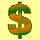## I want to be millionnaire

Knowing that interest rate can make you bankrupt and in debt forever is scary, isn't it? In this section, you will learn to reverse the process. How to use interest rate such that you can become a millionaire.

Of course, the reverse of a loan is an investment.

Say, you are willing to invest \$20 every month in a stock or a bond that on average the growth is about 1% a month. My question is simple: when are you going to become a millionaire? In how many years you will be able to accomplish your goal to become a millionaire?

If you are in doubt, you may say: is it possible to become a millionaire simply investing only \$20 a month?

(If you are impatient, turn to the next page to know the answer.)

I will give you the formula (to become rich J).

Here are the two formulas to find the answer.

Your investment at time t+1 = Your investment at time t + The interest of your investment at time t + Your monthly investment

The interest of your investment at time t = Interest rate * Your investment at time t

If you have read the previous section about loan, the above formula is very similar. The only two differences are:
1. I change the word "loan" and "payment" into "investment"
2. I change the sign from negative payment into positive monthly investment.

In how many months you will be able to pay \$1000 loan at 1% interest rate per month, if you pay \$20 a month?

Again, I do not like long words, thus let us simplify the formula above. Suppose we give notation as follow
V(t) = Your investment at time t
V(t+1) = Your investment at time t+1
k = Interest rate
R(t) = The interest of your investment at time t

The two formulas above can be written as simple as
V(t+1) = V(t) + R(t) + I
R(t) = k * V(t)

Now we can further simplify the two formulas above into a single formula by substituting the second formula into the first formula.
V(t+1) = V(t) + k * V(t) + I

In the right hand side, you can see two terms contain L(t), which we can combine into our last formula

 V(t+1) = (1 + k) * V(t) + I

In words,

 Your investment at time t+1 = (1 + Interest rate ) * Your investment at time t � Your monthly investment

In the beginning, at time t=0, you have zero investment. Thus, V(0) = \$0.

In the first month, your investment will become
V(t+1) = V(t) + k * V(t) + I
V(1) = V(0) + 1% * V(0) - P
V(1) = \$0 + 1% * \$0 + \$20
V(1) = \$0 + \$0 + \$20
V(1) = \$20

In the second month, your investment will become
V(t+1) = V(t) + k * V(t) + I
V(2) = V(1) + 1% * V(1) - P
V(2) = \$20 + 1% * \$20 + \$20
V(2) = \$20 + \$0.20 + \$20
V(2) = \$40.20

It seems not so much in the beginning. But let us see.

Computing manually like this would be cumbersome. We need better tool to compute. Let use spreadsheet (like Microsoft Excel, Google Spreadsheet, etc.) to compute. If you are too lazy to make this spreadsheet on your own, simply donate to Revoledu.Com and you will get the spreadsheet and the PDF version of this tutorial.

First you type the three inputs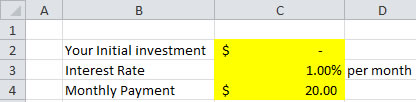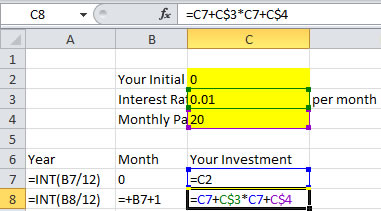After that you copy cell A8:C8 down until A642:C642.

Next, we do the charting to show when your investment will reach \$,1000,000.

In how many months (or years) you will be able to accomplish your goal to become a millionaire?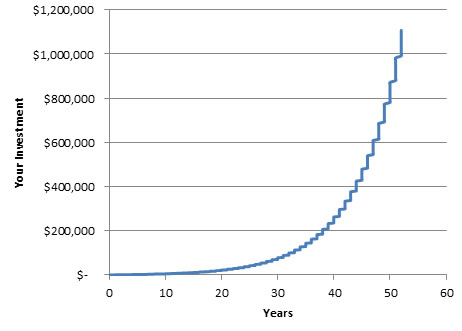To see more detail, look at the chart and the spreadsheet on when your investment will be more than a million dollar.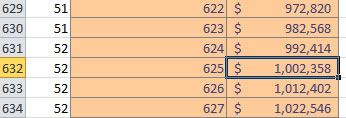You will be able to pay reach your 1 million goal in 625 months, or 52 years.

That is why you need to start early in your investment. At least, start to educate your children to invest.